|

# 为众设计|2017北京751国际设计节强势登陆

9月27日，第七届北京国际设计周751国际设计节于751D PARK北京时尚设计广场拉开帷幕。在9月28日－10月6日为期9天的展览期间内，设计节将呈现百余场精彩纷呈的国内外优质展览与大师论坛项目，并通过新奇有趣的互动体验活动，全面展示“包容性设计”这一设计理念下的国际及本土设计新趋势。开幕式当晚，751D PARK创意产业办公室主任严明丹主持了本届751国际设计节开幕盛会，北京市文资办副主任李小明先生、电控总经理赵炳弟先生、荷兰公使Bas Pulles先生、正东集团总经理张翼先生出席现场。

本届设计节以“为众设计(Inspired by Uniqueness)”为主题，“包容性设计”贯穿整体设计节，旨在探讨如何让设计与社会再次接轨，容纳更广大的群众需求，关心各类群体，以“设计”改变生活现状、提升生活品质，秉持“因不同而共同”的思维，让“包容”成为人性的温度，重新唤醒设计为大众服务的使命。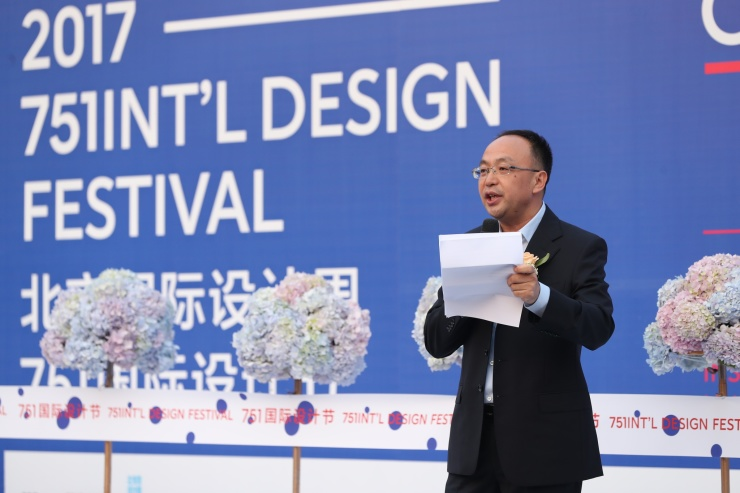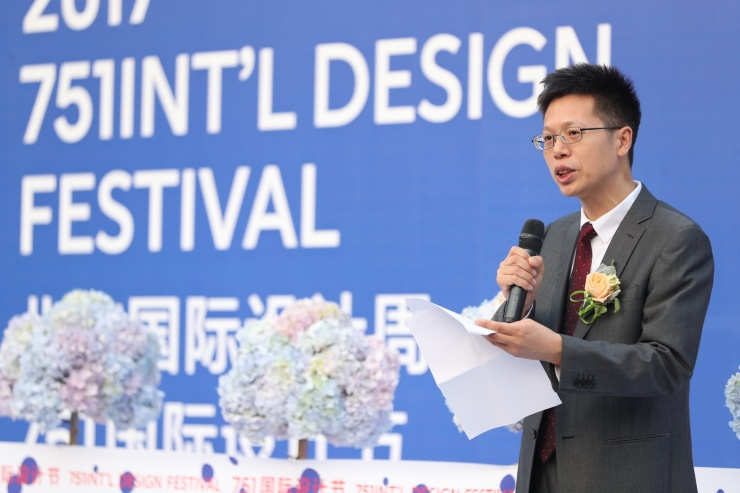2011年至今，751国际设计节已成功举办六届，吸引来自荷兰、英国、意大利、法国、丹麦、美国、西班牙、韩国等10余个国家和地区的数百家品牌参展商前来设展，覆盖面超过2万平米，内容涉及建筑、服装、新材料、工业设计、数字科技、儿童设计教育、家具、食品设计、书籍出版等领域。

【多元展览，洞悉设计内核】

本届751国际设计节展览版块涵盖了精选主题展、互动体验展、多元国际展三大领域。精选主题展中，清华大学与悉尼科技大学合作，以“医、食、住、行”为主题，共同探索“包容性设计”的人文社会价值。

互动体验展中，视觉艺术家丁东将于动力广场呈现由时间、空间与光组成的空间视觉装置展《虚无7.0》，通过与各领域艺术家的跨界合作，开启一段对未知的探索之旅。大型互动体验展《不便利店》将设置包括视觉障碍、听觉障碍、肢体障碍等关卡，让参与者从日常生活的方方面面切身感受不同人群的需求，体会“包容性设计”的人性温度。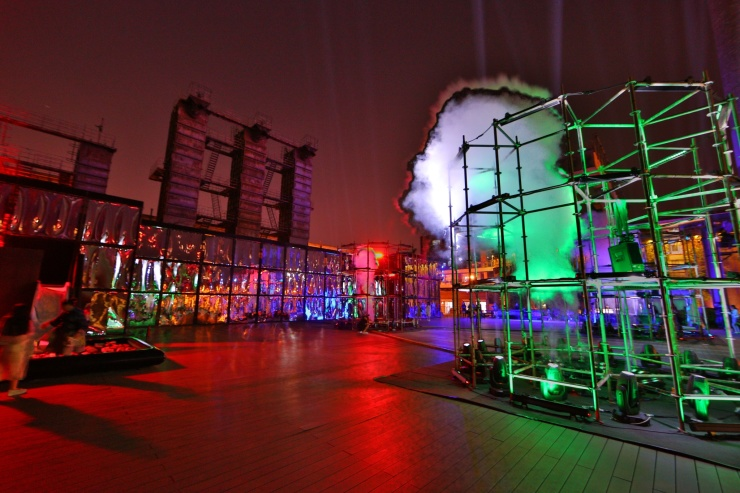多元文化展版块将分别带来以“手工，设计与家居”为主题的《北欧木居－手工、设计与木居展》、与荷兰大使馆联合呈现的《荷兰建筑 & 你》、《荷兰最美的书2016》、《百年荷兰风格派的回顾与致敬》主题展、由澳大利亚大使馆推出的《澳大利亚青年设计奖作品展》，以及奥迪携手国内外优秀设计师、学者、专家一同带来的《未来异位面》，探索新材料科技如何影响人们对于产品及周边环境的前摄观念，从感觉改变感知。【大师论坛，探索设计更多的可能性】

今年751国际设计节将分别以“为众设计：探索设计的边界”、“城市中的人文设计”、“老字号品牌文化复兴”、“为生活提案”为主题，带来四场大师论坛。以包容与尊重的态度，重新体悟设计的价值。更有十一场专题论坛，共同探讨城市更新、未来的公共空间等前沿设计议题。

【用心“玩” 的活动，让设计融入大众文化】

设计私房课、设计市集作为751国际设计节的经典互动项目，将延续往年热潮。此次设计私房课特别邀请“大雨陶艺”，挑选全国各地最自然的泥土和完美的釉料精心设置了“釉下手绘课”和“陶瓷捏雕刻课”。 “POP UP Crossover®创意市集” 、“乐天陶社创意市集”与“BLACKBOOM 黑潮创意嘉年华”三大设计市集也将在十一期间精彩登场。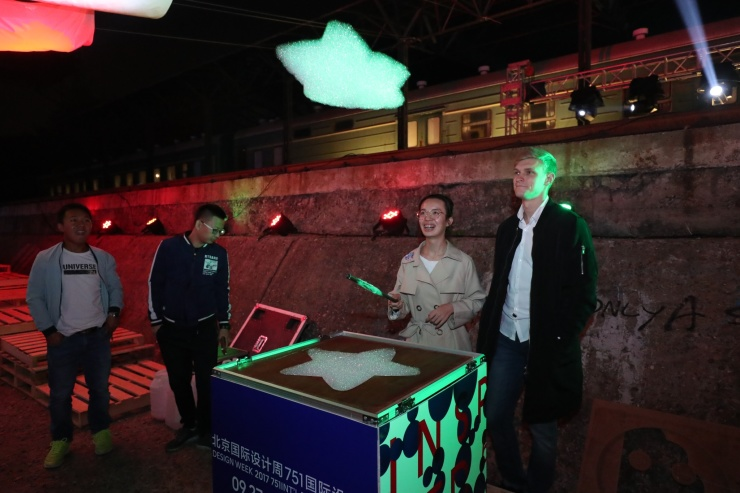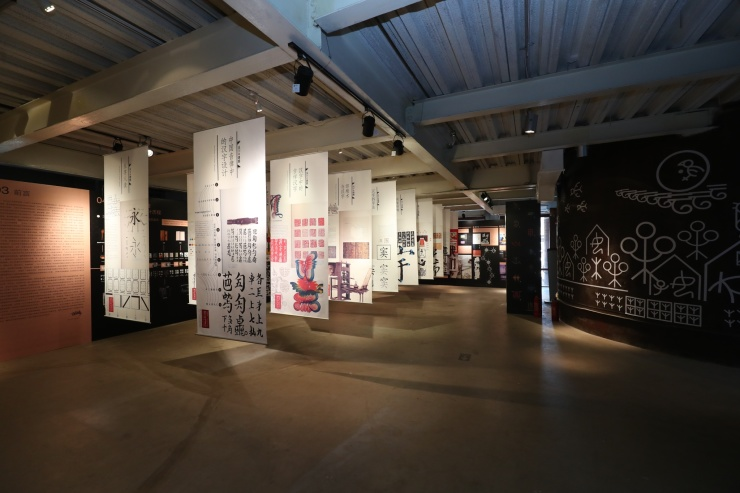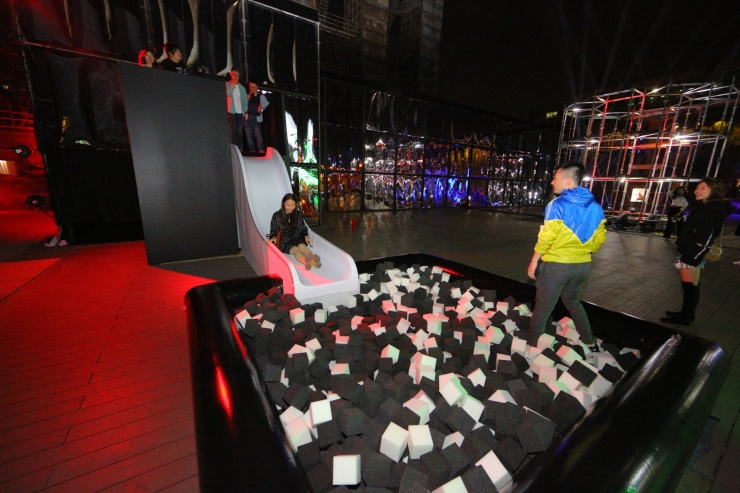作为本届751国际设计节最大的互动亮点“LIFE IS A BEACH”751沙滩电音派对将在751D PARK煤池子震撼开幕，届时北京先锋电子乐厂牌Pajnasonic能量的最强音将会贯穿9月30日－10月4日的每一个夜晚，为公众带来独一无二的沉浸式体验。

【烟囱精灵，最暖心的吉祥物】

本届751国际设计节组委会以“包容性设计”为主线，呼应主题推出了官方吉祥物“烟囱精灵”。它的诞生也正式宣告751国际设计节拥有了自己的卡通形象--大囱&烟宝。设计节期间，除了限量贩售的绒毛玩具，园区内还将不定时现身大型玩偶与大众互动。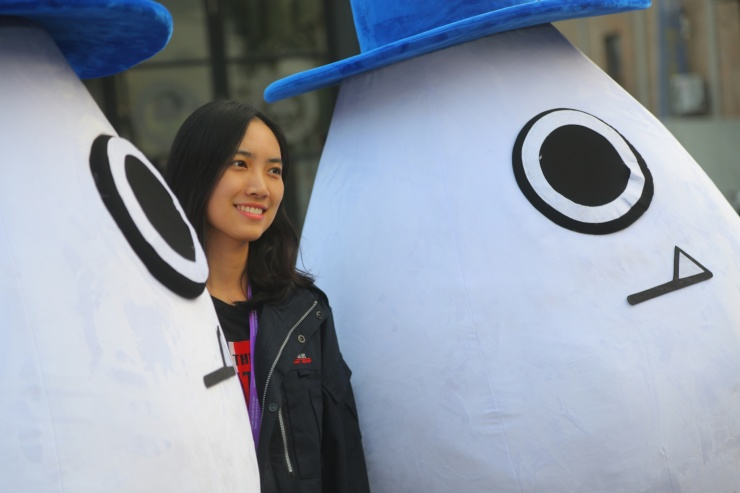2017北京751国际设计节在兼顾学术性和商业性的同时，也从观展体验出发，考虑大众需求，寻求精英化与大众化的平衡，增强设计与广大群众的关联性。751国际设计节作为启发者，旨在推动中国设计的发展，让设计为大众服务。

`声明：本文由入驻焦点开放平台的作者撰写，除焦点官方账号外，观点仅代表作者本人，不代表焦点立场错误信息举报电话： 400-099-0099，邮箱：jubao@vip.sohu.com，或点此进行意见反馈，或点此进行举报投诉。`A B C D E F G H J K L M N P Q R S T W X Y Z
A - B - C - D - E
• A
• 鞍山
• 安庆
• 安阳
• 安顺
• 安康
• 澳门
• B
• 北京
• 保定
• 包头
• 巴彦淖尔
• 本溪
• 蚌埠
• 亳州
• 滨州
• 北海
• 百色
• 巴中
• 毕节
• 保山
• 宝鸡
• 白银
• 巴州
• C
• 承德
• 沧州
• 长治
• 赤峰
• 朝阳
• 长春
• 常州
• 滁州
• 池州
• 长沙
• 常德
• 郴州
• 潮州
• 崇左
• 重庆
• 成都
• 楚雄
• 昌都
• 慈溪
• 常熟
• D
• 大同
• 大连
• 丹东
• 大庆
• 东营
• 德州
• 东莞
• 德阳
• 达州
• 大理
• 德宏
• 定西
• 儋州
• 东平
• E
• 鄂尔多斯
• 鄂州
• 恩施
F - G - H - I - J
• F
• 抚顺
• 阜新
• 阜阳
• 福州
• 抚州
• 佛山
• 防城港
• G
• 赣州
• 广州
• 桂林
• 贵港
• 广元
• 广安
• 贵阳
• 固原
• H
• 邯郸
• 衡水
• 呼和浩特
• 呼伦贝尔
• 葫芦岛
• 哈尔滨
• 黑河
• 淮安
• 杭州
• 湖州
• 合肥
• 淮南
• 淮北
• 黄山
• 菏泽
• 鹤壁
• 黄石
• 黄冈
• 衡阳
• 怀化
• 惠州
• 河源
• 贺州
• 河池
• 海口
• 红河
• 汉中
• 海东
• 怀来
• I
• J
• 晋中
• 锦州
• 吉林
• 鸡西
• 佳木斯
• 嘉兴
• 金华
• 景德镇
• 九江
• 吉安
• 济南
• 济宁
• 焦作
• 荆门
• 荆州
• 江门
• 揭阳
• 金昌
• 酒泉
• 嘉峪关
K - L - M - N - P
• K
• 开封
• 昆明
• 昆山
• L
• 廊坊
• 临汾
• 辽阳
• 连云港
• 丽水
• 六安
• 龙岩
• 莱芜
• 临沂
• 聊城
• 洛阳
• 漯河
• 娄底
• 柳州
• 来宾
• 泸州
• 乐山
• 六盘水
• 丽江
• 临沧
• 拉萨
• 林芝
• 兰州
• 陇南
• M
• 牡丹江
• 马鞍山
• 茂名
• 梅州
• 绵阳
• 眉山
• N
• 南京
• 南通
• 宁波
• 南平
• 宁德
• 南昌
• 南阳
• 南宁
• 内江
• 南充
• P
• 盘锦
• 莆田
• 平顶山
• 濮阳
• 攀枝花
• 普洱
• 平凉
Q - R - S - T - W
• Q
• 秦皇岛
• 齐齐哈尔
• 衢州
• 泉州
• 青岛
• 清远
• 钦州
• 黔南
• 曲靖
• 庆阳
• R
• 日照
• 日喀则
• S
• 石家庄
• 沈阳
• 双鸭山
• 绥化
• 上海
• 苏州
• 宿迁
• 绍兴
• 宿州
• 三明
• 上饶
• 三门峡
• 商丘
• 十堰
• 随州
• 邵阳
• 韶关
• 深圳
• 汕头
• 汕尾
• 三亚
• 三沙
• 遂宁
• 山南
• 商洛
• 石嘴山
• T
• 天津
• 唐山
• 太原
• 通辽
• 铁岭
• 泰州
• 台州
• 铜陵
• 泰安
• 铜仁
• 铜川
• 天水
• 天门
• W
• 乌海
• 乌兰察布
• 无锡
• 温州
• 芜湖
• 潍坊
• 威海
• 武汉
• 梧州
• 渭南
• 武威
• 吴忠
• 乌鲁木齐
X - Y - Z
• X
• 邢台
• 徐州
• 宣城
• 厦门
• 新乡
• 许昌
• 信阳
• 襄阳
• 孝感
• 咸宁
• 湘潭
• 湘西
• 西双版纳
• 西安
• 咸阳
• 西宁
• 仙桃
• 西昌
• Y
• 运城
• 营口
• 盐城
• 扬州
• 鹰潭
• 宜春
• 烟台
• 宜昌
• 岳阳
• 益阳
• 永州
• 阳江
• 云浮
• 玉林
• 宜宾
• 雅安
• 玉溪
• 延安
• 榆林
• 银川
• Z
• 张家口
• 镇江
• 舟山
• 漳州
• 淄博
• 枣庄
• 郑州
• 周口
• 驻马店
• 株洲
• 张家界
• 珠海
• 湛江
• 肇庆
• 中山
• 自贡
• 资阳
• 遵义
• 昭通
• 张掖
• 中卫

1室1厅1厨1卫1阳台

1
2
3
4
5

0
1
2

1

1

0
1
2
3报名成功，资料已提交审核A B C D E F G H J K L M N P Q R S T W X Y Z
A - B - C - D - E
• A
• 鞍山
• 安庆
• 安阳
• 安顺
• 安康
• 澳门
• B
• 北京
• 保定
• 包头
• 巴彦淖尔
• 本溪
• 蚌埠
• 亳州
• 滨州
• 北海
• 百色
• 巴中
• 毕节
• 保山
• 宝鸡
• 白银
• 巴州
• C
• 承德
• 沧州
• 长治
• 赤峰
• 朝阳
• 长春
• 常州
• 滁州
• 池州
• 长沙
• 常德
• 郴州
• 潮州
• 崇左
• 重庆
• 成都
• 楚雄
• 昌都
• 慈溪
• 常熟
• D
• 大同
• 大连
• 丹东
• 大庆
• 东营
• 德州
• 东莞
• 德阳
• 达州
• 大理
• 德宏
• 定西
• 儋州
• 东平
• E
• 鄂尔多斯
• 鄂州
• 恩施
F - G - H - I - J
• F
• 抚顺
• 阜新
• 阜阳
• 福州
• 抚州
• 佛山
• 防城港
• G
• 赣州
• 广州
• 桂林
• 贵港
• 广元
• 广安
• 贵阳
• 固原
• H
• 邯郸
• 衡水
• 呼和浩特
• 呼伦贝尔
• 葫芦岛
• 哈尔滨
• 黑河
• 淮安
• 杭州
• 湖州
• 合肥
• 淮南
• 淮北
• 黄山
• 菏泽
• 鹤壁
• 黄石
• 黄冈
• 衡阳
• 怀化
• 惠州
• 河源
• 贺州
• 河池
• 海口
• 红河
• 汉中
• 海东
• 怀来
• I
• J
• 晋中
• 锦州
• 吉林
• 鸡西
• 佳木斯
• 嘉兴
• 金华
• 景德镇
• 九江
• 吉安
• 济南
• 济宁
• 焦作
• 荆门
• 荆州
• 江门
• 揭阳
• 金昌
• 酒泉
• 嘉峪关
K - L - M - N - P
• K
• 开封
• 昆明
• 昆山
• L
• 廊坊
• 临汾
• 辽阳
• 连云港
• 丽水
• 六安
• 龙岩
• 莱芜
• 临沂
• 聊城
• 洛阳
• 漯河
• 娄底
• 柳州
• 来宾
• 泸州
• 乐山
• 六盘水
• 丽江
• 临沧
• 拉萨
• 林芝
• 兰州
• 陇南
• M
• 牡丹江
• 马鞍山
• 茂名
• 梅州
• 绵阳
• 眉山
• N
• 南京
• 南通
• 宁波
• 南平
• 宁德
• 南昌
• 南阳
• 南宁
• 内江
• 南充
• P
• 盘锦
• 莆田
• 平顶山
• 濮阳
• 攀枝花
• 普洱
• 平凉
Q - R - S - T - W
• Q
• 秦皇岛
• 齐齐哈尔
• 衢州
• 泉州
• 青岛
• 清远
• 钦州
• 黔南
• 曲靖
• 庆阳
• R
• 日照
• 日喀则
• S
• 石家庄
• 沈阳
• 双鸭山
• 绥化
• 上海
• 苏州
• 宿迁
• 绍兴
• 宿州
• 三明
• 上饶
• 三门峡
• 商丘
• 十堰
• 随州
• 邵阳
• 韶关
• 深圳
• 汕头
• 汕尾
• 三亚
• 三沙
• 遂宁
• 山南
• 商洛
• 石嘴山
• T
• 天津
• 唐山
• 太原
• 通辽
• 铁岭
• 泰州
• 台州
• 铜陵
• 泰安
• 铜仁
• 铜川
• 天水
• 天门
• W
• 乌海
• 乌兰察布
• 无锡
• 温州
• 芜湖
• 潍坊
• 威海
• 武汉
• 梧州
• 渭南
• 武威
• 吴忠
• 乌鲁木齐
X - Y - Z
• X
• 邢台
• 徐州
• 宣城
• 厦门
• 新乡
• 许昌
• 信阳
• 襄阳
• 孝感
• 咸宁
• 湘潭
• 湘西
• 西双版纳
• 西安
• 咸阳
• 西宁
• 仙桃
• 西昌
• Y
• 运城
• 营口
• 盐城
• 扬州
• 鹰潭
• 宜春
• 烟台
• 宜昌
• 岳阳
• 益阳
• 永州
• 阳江
• 云浮
• 玉林
• 宜宾
• 雅安
• 玉溪
• 延安
• 榆林
• 银川
• Z
• 张家口
• 镇江
• 舟山
• 漳州
• 淄博
• 枣庄
• 郑州
• 周口
• 驻马店
• 株洲
• 张家界
• 珠海
• 湛江
• 肇庆
• 中山
• 自贡
• 资阳
• 遵义
• 昭通
• 张掖
• 中卫• 手机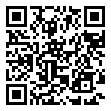• 分享
• 设计
免费设计
• 计算器
装修计算器
• 入驻
合作入驻
• 联系
联系我们
• 置顶
返回顶部